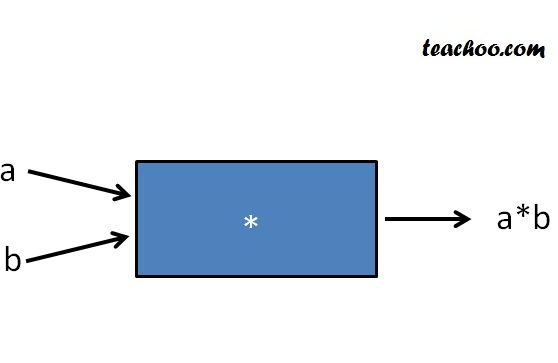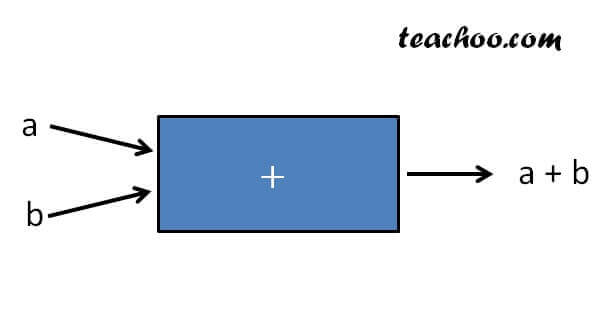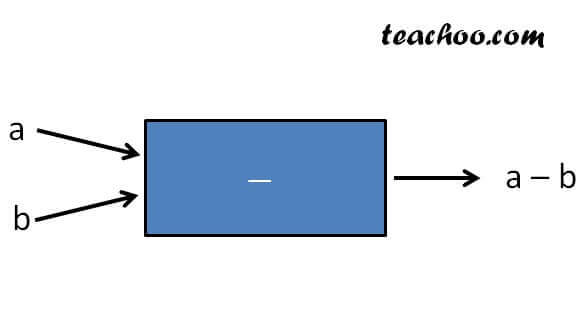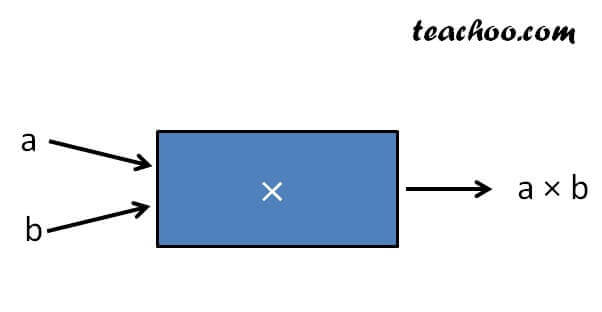Binary Operations - Definition

Chapter 1 Class 12 Relation and Functions
Concept wise

In binary operations,

we take two numbers and get one number.

All the numbers are in the same set.

For binary operation

* : A × A → AHere,

a, b and a*b all lie in same set A

Let's look at some examples

## Sum is a binary operation in R

In R (Set of real numbers),

Sum is a binary operation

Let’s take an example

For

+ : R × R R

where (a, b) → a + bFor every real number a & b,

a + b is also a real number.

Hence, + is a binary operation on R

## Subtraction is a binary operation in R

In R (Set of real numbers),

Subtraction is a binary operation

Let’s take an example

For

– : R × R R

where (a, b) → a – bFor every real number a & b,

a – b is also a real number.

Hence, – is a binary operation on R

## Multiplication is a binary operation in R

In R (Set of real numbers),

Multiplication is a binary operation

Let’s take an example

For

× : R × R R

where (a, b) → a × bFor every real number a & b,

a × b is also a real number.

Hence,  × is a binary operation on R

## Division is NOT binary operation in R

In R (Set of real numbers),

Division is not a binary operation

For

÷: R × R R

where (a, b) → a ÷ b

Here, a & b are real numbers

a ÷ b = a/b

Let a = 2 & b = 0

a/b = 2/0 = Not defined

Hence, ÷ is not a binary operation on R

Learn in your speed, with individual attention - Teachoo Maths 1-on-1 Class